# How to Sum the bottom 5 or n Values in a column in Excel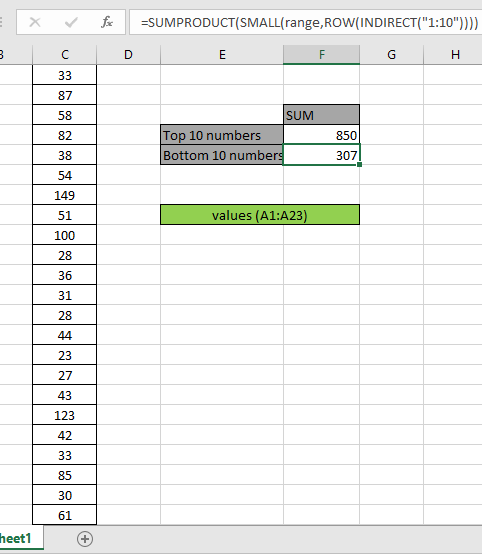In this article, we will learn How to Sum the bottom 5 or n Values in a column in Excel.

Scenario:

In simple words, while working with data numbers, sometimes given a condition i.e. when we need to get the sum of the numbers of the bottom or lowest 5 numbers from the array or bottom of the array. You can perform the solution to this problem easily using the excel functions as explained below.

Sum of Bottom N values

How to solve the problem?

For this article we will be required to use the SUMPRODUCT function. Now we will make a formula out of these functions. Here we are given a range and we need to bottom 5 values in range and get the sum of the values.

Generic formula:

 = SUMPRODUCT ( SMALL ( range, { 1 , 2 , .... ,n } } )

range : range of values

Values : numbers separated using the commas like if you wish to find the bottom 3 values, use { 1 , 2 , 3 }.

Example:

All of these might be confusing to understand. So, let's test this formula via running it on the example shown below.

Here we have a range of values from A1:A23.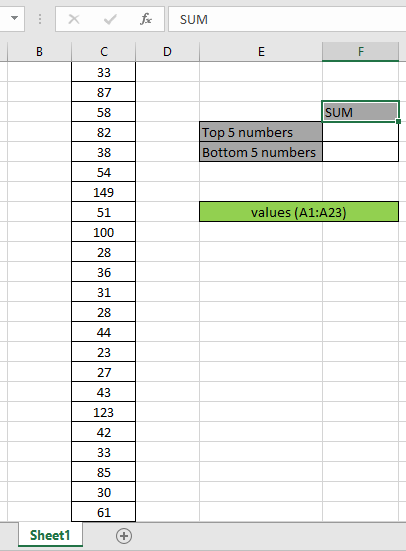Here the range is given as using the named range excel tool.

Firstly,  we need to find the bottom Five values using the SMALL function and then sum operation be performed over those 5 values. Now we will use the following formula to get the sum

Use the Formula:

 = SUMPRODUCT ( SMALL ( range, { 1 , 2 , 3 , 4 , 5 } ) )

Explanation:

• SMALL function returns the bottom 5 numerical values and returns the array to the SUMPRODUCT function.

= SUMPRODUCT ( { 23 , 27 , 28 , 28 , 30 } ) )

• SUMPRODUCT function gets the array of bottom 5 values, which has an array of bottom 5 numbers returns the SUM of those numbers.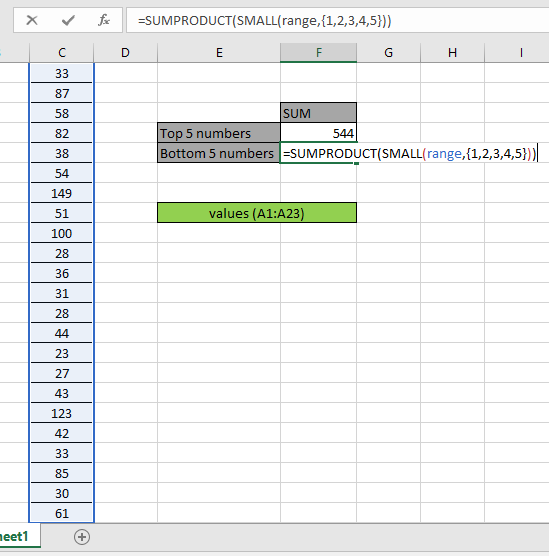Here the range is given as the named range. Press Enter to get the SUM of the bottom 5 numbers.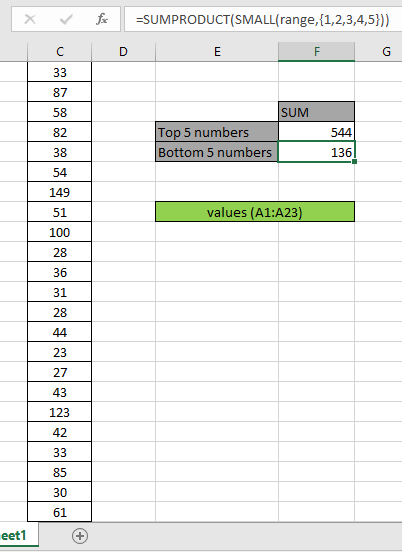As you can see in the above snapshot that sum is 136. The sum of the values 23 + 27 + 28 + 28 + 30 = 136.

The above process is used to calculate the sum of a few numbers from the bottom. But to calculate for n (large) number of values in a long range.

Use the formula:

 = SUMPRODUCT ( SMALL ( range, ROW ( INDIRECT ( "1:10" ) )

Here we generate sum of bottom 10 values via getting an array of 1 to 10 { 1 ; 2 ; 3 ; 4 ; 5 ; 6 ; 7 ; 8 ; 9 ; 10 } using the ROW & INDIRECT Excel functions.Here we got the sum of the bottom 10 numbers which results in 307.

Sum of TOP N values

How to solve the problem?

For this article we will be required to use the SUMPRODUCT function. Now we will make a formula out of these functions. Here we are given a range and we need to top 5 values in range and get the sum of the values.

Generic formula:

 = SUMPRODUCT ( LARGE ( range, { 1 , 2 , .... ,n } } )

range : range of values

Values : numbers separated using the commas like if you wish to find the top 3 values, use { 1 , 2 , 3 }.

Example:

All of these might be confusing to understand. So, let's test this formula via running it on the example shown below.

Here we have a range of values from A1:A23.Here the range is given as using the named range excel tool.

Firstly,  we need to find the top Five values using the LARGE function and then sum operation be performed over those 5 values. Now we will use the following formula to get the sum

Use the Formula:

 = SUMPRODUCT ( LARGE ( range, { 1 , 2 , 3 , 4 , 5 } ) )

Explanation:

• LARGE function returns the top 5 numerical values and return the array to the SUMPRODUCT function.

= SUMPRODUCT ( { 149 , 123 , 100 , 87 , 85 } ) )

• SUMPRODUCT function gets the array of top 5 values, which has an array of top 5 numbers returns the SUM of those numbers.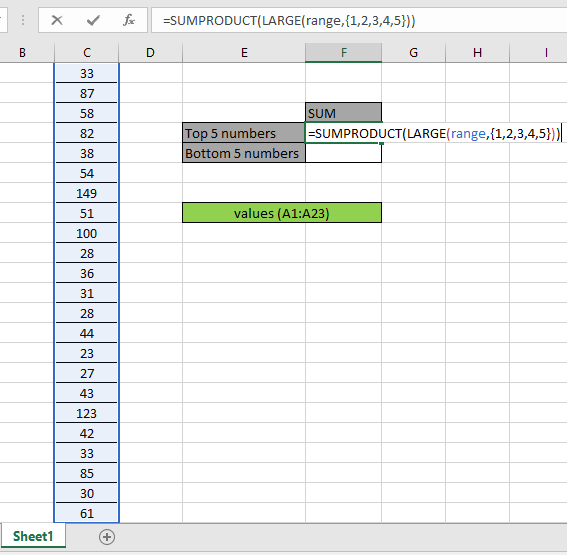Here the range is given as the named range. Press Enter to get the SUM of top 5 numbers.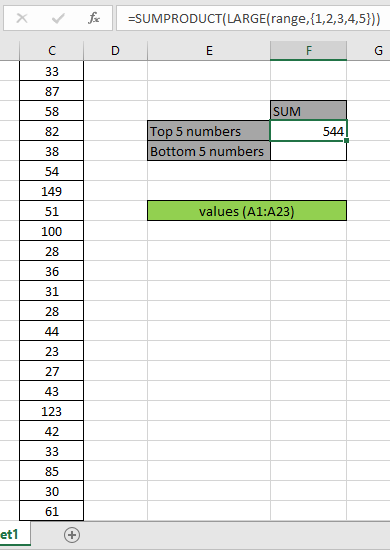As you can see in the above snapshot that sum is 544. The sum of the values 149 + 123 + 100 + 87 + 85 = 544.

The above process is used to calculate the sum of a few numbers from the top. But to calculate for n (large) number of values in a long range.

Use the formula:

 = SUMPRODUCT ( LARGE ( range, ROW ( INDIRECT ( "1:10" ) )

Here we generate sum of top 10 values via getting an array of 1 to 10 { 1 ; 2 ; 3 ; 4 ; 5 ; 6 ; 7 ; 8 ; 9 ; 10 } using the ROW & INDIRECT Excel functions.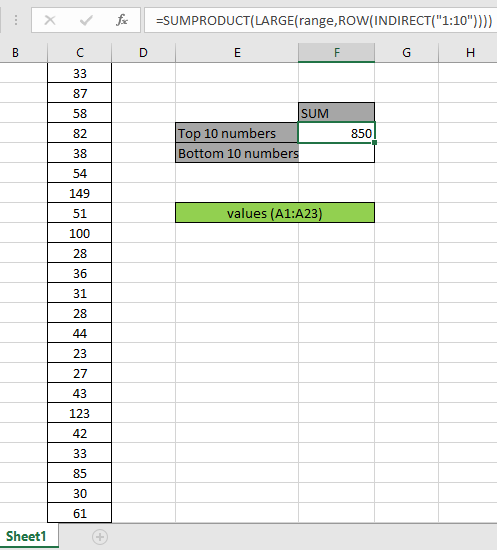Here we got the sum of the top 10 numbers { 149 ; 123 ; 100 ; 87 ; 85 ; 82 ; 61 ; 58 ; 54 ; 51 }) which results in 850.

Here are some observational notes shown below.

Notes:

1. The formula only works with numbers.
2. The formula works only when there are no duplicates in the lookup table
3. The SUMPRODUCT function considers non - numeric values ( like text abc ) and error values ( like #NUM! , #NULL! )  as null values.
4. The SUMPRODUCT function considers logic value TRUE as 1 and False as 0.
5. The argument array must be of the same length else the function.

Hope this article about How to Sum the bottom 5 or n Values in a column in Excel is explanatory. Find more articles on calculating values and related Excel formulas here. If you liked our blogs, share it with your friends on Facebook. And also you can follow us on Twitter and Facebook. We would love to hear from you, do let us know how we can improve, complement or innovate our work and make it better for you. Write to us at info@exceltip.com.

Related Articles :

How to use the SUMPRODUCT function in Excel: Returns the SUM after multiplication of values in multiple arrays in excel.

SUM if date is between : Returns the SUM of values between given dates or period in excel.

Sum if date is greater than given date: Returns the SUM of values after the given date or period in excel.

2 Ways to Sum by Month in Excel: Returns the SUM of values within a given specific month in excel.

How to Sum Multiple Columns with Condition: Returns the SUM of values across multiple columns having condition in excel

How to use wildcards in excel : Count cells matching phrases using the wildcards in excel

Popular Articles :

How to use the IF Function in Excel : The IF statement in Excel checks the condition and returns a specific value if the condition is TRUE or returns another specific value if FALSE.

How to use the VLOOKUP Function in Excel : This is one of the most used and popular functions of excel that is used to lookup value from different ranges and sheets.

How to use the SUMIF Function in Excel : This is another dashboard essential function. This helps you sum up values on specific conditions.

How to use the COUNTIF Function in Excel : Count values with conditions using this amazing function. You don't need to filter your data to count specific values. Countif function is essential to prepare your dashboard.

Terms and Conditions of use

The applications/code on this site are distributed as is and without warranties or liability. In no event shall the owner of the copyrights, or the authors of the applications/code be liable for any loss of profit, any problems or any damage resulting from the use or evaluation of the applications/code.